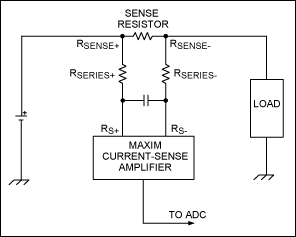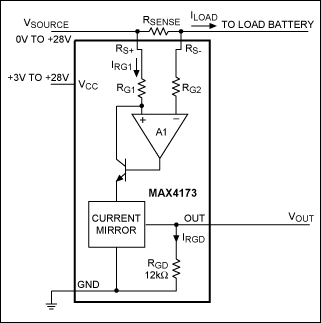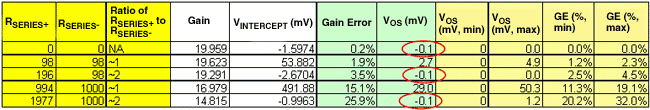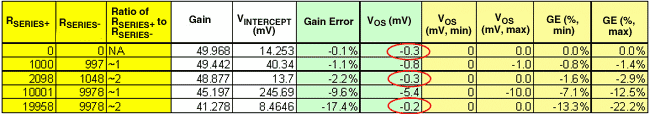# Performance of Current-Sense Amplifiers with Input Series Resistors

### Abstract

This application note explains some performance characteristics of high-side current-sense amplifiers. The article explains how to use these amplifiers with series resistors on the sense inputs. This design approach is especially useful for applications that need to amplify small voltages across a sense resistor on a high-voltage rail, and feed it to a low-voltage ADC or a low-voltage analog control loop. Test data from the MAX4173 and the MAX4372 are presented.

### Introduction

When discussing functional operation, a current-sense amplifier can be considered an instrumentation/differential amplifier with a floating input stage. This means that even when the device is powered from a single-supply with VCC = 3.3V or 5V, it can amplify input differential signals at a common-mode voltage well beyond these power supply rails. The common-mode voltages in a current-sense amplifier can, for example, be up to 28V (MAX4372 and MAX4173) and 76V (MAX4080 and MAX4081).

This performance characteristic of current-sense amplifiers is extremely useful for high-side current-sensing applications that need to amplify small voltages across a sense resistor on a high-voltage rail, and feed it to a low-voltage ADC or a low-voltage analog control loop. In these applications the current-sense signal frequently needs to be filtered at the source, i.e., across the sense resistor. The design could use either a differential filter (Figure 1) to smooth "spiky" load currents and sense voltages, or a common-mode filter (Figure 2) to enhance ESD operation/immunity to common-mode voltage spikes and temporary overvoltages. These filters can be successfully implemented by choosing the right component values. If the wrong component values are selected, however, unplanned input offset voltages and gain errors can be introduced, which compromise circuit performance.Figure 1. Circuit diagram for a differential filter to smooth spiky load currents.Figure 2. Circuit diagram for a common-mode filter to improve immunity to ESD spikes and common-mode overvoltages.

### Determining which Filters to Use

Consider now the MAX4173 current-sense amplifier shown in Figure 3. This device has its sense resistor connected directly to the RS+ and RS- terminals of the chip. The differential voltage across the sense resistor is reproduced across RG1 by internal operational-amplifier function so that ILOAD × RSENSE = VSENSE = IRG1 × RG1. This current (IRG1) is then level-shifted and amplified by an internal current mirror to generate the output current, IRGD. The internal circuit for the MAX4173 implements RGD = 12k and RG1 = 6k.

Therefore,

VOUT = RGD × IRGD = RGD × Gain × IRG1 = RGD × Gain × VSENSE/RG1

As RGD and RG1 are on-chip resistors, their actual values normally vary by as much as ±30% due to semiconductor process variations. However, because it is the ratio of RGD and RG1 that determines the final gain accuracy, the final gain is well controlled and can be easily trimmed during manufacture.Figure 3. Internal functional diagram of the MAX4173.

However, when series resistors are inserted between the RSENSE+ and RSENSE- terminals of a sense resistor, and RS+ and RS- pins of the part to implement differential/common-mode filters (as shown in Figure 1 and Figure 2), the chip behaves as though RG1 and RG2 have been modified. From the above equation, it is apparent that modifying a trimmed RG1 introduces a gain error. Further, since the absolute value of RG1 can vary by as much as ±30%, this gain error can also vary by ±30% and cannot be controlled or predicted between multiple parts. The only way to control this gain error is, therefore, to ensure that the input series resistor, RSERIES+, is small compared to RG1.

Additionally, a mismatch between resistors RG1 and RG2 is "converted" by the device's input bias currents into an input offset voltage. The MAX4173 and MAX4372 data sheets show that bias current IRS- is twice IRS+, and therefore, any resistor in series with RG1 (RSERIES+) should be twice that in series with RG2 (RSERIES-) to cancel the input offset voltage. Similar bias current characteristics are also present for the following current-sense amplifiers: MAX4073, MAX4172, MAX4373–MAX4375 and MAX4376–MAX4378. Therefore, similar techniques can be used to size input resistors for proper differential/common-mode filter design.

### Summary and Proof

To summarize, ideal performance can be obtained from input filters with series resistors between the sense resistor and RS+ and RS- pins if:

1. The series resistor between RSENSE+ and RS+ is kept small with respect to RG1.
2. The series resistor between RSENSE+ and RS+ is twice as big as that between RSENSE- and RS-.
Note, finally, that since RSERIES+ is twice RSERIES-, the common-mode filter capacitors will also need to be suitably scaled to meet desired AC and transient performance objectives.

The bench-test results in Table 1 were obtained with MAX4173T and support the discussion above. The min and max values of VOS were calculated using min and max bias currents from the data sheet; the min and max gain errors were calculated using ±30% of RG1 = 6k.

Table 1. Series Resistor Test Results for the MAX4173Similarly, bench results obtained with MAX4372F are shown below in Table 2 (RG1 = 100k).

Table 2. Series Resistor Test Results for the MAX4372The derivation of calculated min and max gain errors and min-max VOS is shown below.

Old Gain

= Constant × RGD/RG1 = 20 (for T-version of MAX4173)
New Gain
= Constant × RGD/RG1new; RG1new = RG1 + RSERIES+
= Old Gain × RG1/RG1new
= 20 × RG1/(RG1 + RSERIES+)
Gain Error
= (20 - New Gain)/20%
= RSERIES+/(RG1 + RSERIES+)
Min Gain Error
= RSERIES+/(1.3 × RG1 + RSERIES+)
Max Gain Error
= RSERIES+/(0.7 × RG1 + RSERIES+)
RG1 = 6k for MAX4173

VOS = IBIAS2 × RG2new - IBIAS1 × RG1new

= IBIAS1 × ((2 × RSERIES-) - RSERIES+); where IBIAS2 = 2 × IBIAS1

IBIAS1(min) = 0
IBIAS1(max) = 50µA for MAX4173

A similar article first appeared on the Planet Analog website on September 26, 2007.